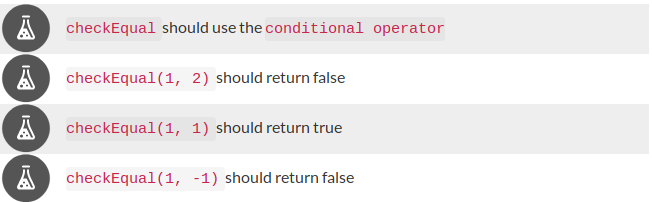# FindError_in_Freecodecamp

1>-1 is true
Freecodecamp tutorial is saying False over here,
so how can I pass this test?

I found in Javascript subject in ternary operators

function checkEqual(a, b) {

return a >== b ? true : false;

}

checkEqual(1, 2);

checkEqual(1, 1);

checkEqual(1,-1);

its in Basic Javascript course and in Ternary operators!
freecodecamp|652x202If this is your code, you are using `>==` which is not an operator you can use

You can use `>=` which is “greater than or equal to”, or `===` which is “strictly equal”

I need to check if they are equal. Not if one is bigger than the other. As such `checkEqual(1, -1)` should correctly return `false`

1 Like